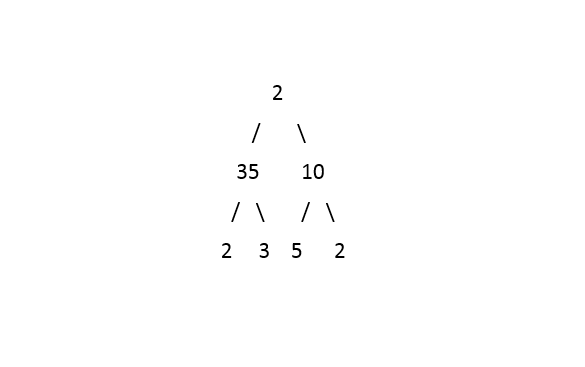New update is available. Click here to update.

# Left View Of Binary Tree

Last Updated: 31 Oct, 2017
Difficulty: Moderate

## PROBLEM STATEMENT

#### Given a binary tree. Print the Left View of the Tree.

##### Example :
``````If the input tree is as depicted in the picture:
````````````The Left View of the tree will be:  2 35 2
``````
##### Input format :
``````Elements in the level order form. The input consists of values of nodes separated by a single space in a single line. In case a node is null, we take -1 in its place.

For example, the input for the tree depicted in the below image would be :
````````````1
2 3
4 -1 5 6
-1 7 -1 -1 -1 -1
-1 -1
``````

#### Explanation :

``````Level 1 :
The root node of the tree is 1

Level 2 :
Left child of 1 = 2
Right child of 1 = 3

Level 3 :
Left child of 2 = 4
Right child of 2 = null (-1)
Left child of 3 = 5
Right child of 3 = 6

Level 4 :
Left child of 4 = null (-1)
Right child of 4 = 7
Left child of 5 = null (-1)
Right child of 5 = null (-1)
Left child of 6 = null (-1)
Right child of 6 = null (-1)

Level 5 :
Left child of 7 = null (-1)
Right child of 7 = null (-1)

The first not-null node (of the previous level) is treated as the parent of the first two nodes of the current level. The second not-null node (of the previous level) is treated as the parent node for the next two nodes of the current level and so on.

The input ends when all nodes at the last level are null (-1).
``````
##### Note :
``````The above format was just to provide clarity on how the input is formed for a given tree.

The sequence will be put together in a single line separated by a single space. Hence, for the above-depicted tree, the input will be given as:

1 2 3 4 -1 5 6 -1 7 -1 -1 -1 -1 -1 -1
``````
##### Output Format :
``````The only line of output will print the data of in the Left View of the Tree separated by a single space.
``````
##### Constraints :
``````0 <= N <= 10^5
1 <= data <= 10^5

Where ‘N’ is the total number of nodes in the binary tree, and 'data' is the value of the binary tree node.

Time limit: 1sec
``````## Approach 1

This problem can be solved through recursion.We will maintain max_level variable which will keep track of maxLevel and will pass current level in recursion as argument. Whenever we see a node whose current level is more than maxLevel then we will print that node as that will be first node for that current level. Also update maxLevel with current level.